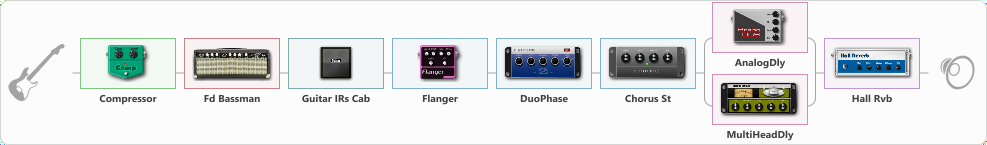# Duo Phase Tone

Discussion in 'ToneLib-GFX presets' started by truss van halen, Dec 12, 2020.

1. ### truss van halenWell-Known Member

Duo Phase Tone

Preset name: Duo Phase tone

Effects chain:Effect: "Compressor" (Dynamics / Filter), active - "yes"
{
"Sense" = 31
"Level" = 49
}

Effect: "Fd Bassman" (Amp simulators), active - "yes"
{
"Gain" = 30
"Bass" = 49
"Middle" = 54
"Treble" = 59
"Presence" = 38
"Master" = 60
"Level (dB)" = 2
}

Effect: "Guitar IRs Cab" (Cabinets), active - "yes"
{
"Model" = Fender Twin Rev (2x12")
"Mic Position" = Edge
"Mic Distance" = Near
"Low Cut (Hz)" = 60
"Hi Cut (kHz)" = 20.0
"Mix" = 100
"Level (dB)" = 0
}

Effect: "Flanger" (Modulation / Sfx), active - "yes"
{
"Speed" = 0.8
"Depth" = 71
"Reso" = 35
"Center" = 7.7
"Offset" = 0.0
"Mix" = 75
"Reset" = On
}

Effect: "DuoPhase" (Modulation / Sfx), active - "yes"
{
"Speed A" = 4.1
"Speed B" = 7.2
"Depth" = 76
"Reso" = 30
"Mode" = ParaSncPhase
}

Effect: "Chorus St" (Modulation / Sfx), active - "yes"
{
"Speed" = 3.2
"Depth" = 51
"Center" = 6.4
"Mix" = 100
}

Effect: "Splitter" (Dynamics / Filter), active - "yes"
{
"A-Bypass" = Off
"A-Pan" = 0
"A-Level" = 55
"B-Bypass" = Off
"B-Pan" = 0
"B-Level" = 55

'A' branch:
{

Effect: "AnalogDly" (Delay), active - "yes"
{
"Time" = 644
"Feedback" = 42
"Tone" = 65
"Mix" = 46
}
}
'B' branch:
{

Effect: "MultiHeadDly" (Delay), active - "yes"
{
"Time" = 205
"Feedback" = 40
"Tone" = 58
"MODE" = Rhythm X-0-X-X
"Mix" = 60
}
}
}

Effect: "Hall Rvb" (Reverberation), active - "yes"
{
"Time" = 9.4
"PreDelay" = 61
"LoDamp" = 49
"HiDamp" = 17
"Mix" = 44
}

Note: You will need to download and install the ToneLib-GFX software to use the preset.

File size:
961 bytes
Views:
2,866